# 1 Introduction

出发角度：倾向于保持重要的结构和属性不变，同时干扰可能不重要的边连接和特征。

自适应数据增强方面：

• 拓扑结构：基于节点中心性度量，突出重要连接；
• 语义信息：对不重要的节点属性添加噪声；

# 2 Method

## 2.1 Framework

框架如下：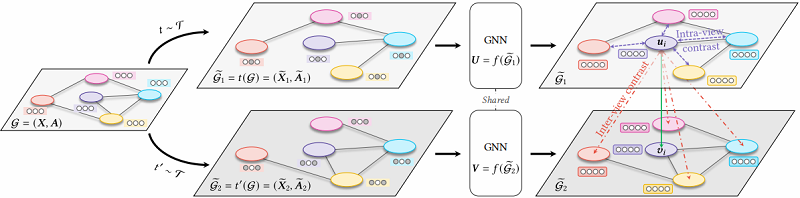形如 GRACE 架构。

算法流程：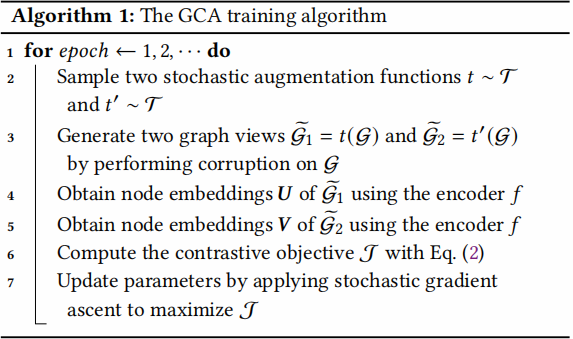编码器

\begin{aligned}\mathrm{GC}_{i}(\boldsymbol{X}, \boldsymbol{A}) &=\sigma\left(\hat{D}^{-\frac{1}{2}} \hat{\boldsymbol{A}} \hat{D}^{-\frac{1}{2}} \boldsymbol{X} \boldsymbol{W}_{i}\right)\quad\quad\quad(12) \\f(\boldsymbol{X}, \boldsymbol{A}) &=\mathrm{GC}_{2}\left(\mathrm{GC}_{1}(\boldsymbol{X}, \boldsymbol{A}), \boldsymbol{A}\right)\quad\quad\quad(13)\end{aligned}

损失函数

$\mathcal{J}=\frac{1}{2 N} \sum\limits _{i=1}^{N}\left[\ell\left(\boldsymbol{u}_{i}, v_{i}\right)+\ell\left(v_{i}, \boldsymbol{u}_{i}\right)\right]\quad\quad\quad(2)$

其中：

$log {\large \frac{e^{\theta\left(u_{i}, v_{i}\right) / \tau}}{\underbrace{e^{\theta\left(\boldsymbol{u}_{i}, \boldsymbol{v}_{i}\right) / \tau}}_{\text {positive pair }}+\underbrace{\sum_{k \neq i} e^{\theta\left(\boldsymbol{u}_{i}, \boldsymbol{v}_{k}\right) / \tau}}_{\text {inter-view negative pairs }}+\underbrace{\sum_{k \neq i} e^{\theta\left(\boldsymbol{u}_{i}, \boldsymbol{u}_{k}\right) / \tau}}_{\text {intra-view negative pairs }}}}\quad\quad\quad(1)$

## 2.2 Adaptive Graph Augmentation

### 2.2.1 Topology-level augmentation

利用 $\text{Eq.3}$ 中的概率从原始边集合中采样一个边子集合

$P\{(u, v) \in \widetilde{\mathcal{E}}\}=1-p_{u v}^{e}\quad\quad\quad(3)$

其中：

• $(u, v) \in \mathcal{E}$；
• $p_{u v}^{e}$ 是删除边 $(u, v)$ 的概率；
• $\widetilde{\mathcal{E}}$ 将作为生成视图的边集合；

分析知： $p_{u v}^{e}$ 应该反映边 $(u, v)$ 的重要性，目的是大概率删除不重要的边，同时保留增强视图中重要的边。

节点中心性量化了节点的重要性，本文为边 $(u, v)$ 定义边中心性 $w_{u v}^{e}$，用于衡量边$(u, v)$ 对两个相连节点的影响。给定节点中心性度量 $\varphi_{c}(\cdot): \mathcal{V} \rightarrow \mathbb{R}^{+}$，将边中心性定义为两个相邻节点中心性得分的均值，即 $w_{u v}^{e}=\left(\varphi_{c}(u)+\varphi_{c}(v)\right) / 2$。在有向图上，只使用尾部节点的中心性，即 $w_{u v}^{e}=\varphi_{c}(v)$，因为边的重要性通常是它们指向的节点。

接下来，根据每条边的中心性值来计算它的概率。由于采用度作为节点中心性这种度量方法在不同数量级上变化差别过大，所以本文首先设置 $s_{u v}^{e}=\log w_{u v}^{e}$ 以缓解具有高度密集连接的节点的影响。然后通过将边中心性的值转换为概率：

$p_{u v}^{e}=\underset{}{\text{min}} \left(\frac{s_{\max }^{e}-s_{u v}^{e}}{s_{\max }^{e}-\mu_{s}^{e}} \cdot p_{e}, \quad p_{\tau}\right)\quad\quad\quad(4)$

其中，$p_{e}$ 是一个控制去除边的总体概率的超参数，$s_{\max }^{e}$ 和 $\mu_{s}^{e}$ 是 $s_{u v}^{e}$ 的最大值和平均值。而 $p_{\tau}<1$ 是一个临界概率（cut-off probability），对于边中心性低（高概率删除）的边，采用 $p_{\tau}$ 删除，对于边中心性低的边（低概率删除），采用 ${\large \frac{s_{\max }^{e}-s_{u v}^{e}}{s_{\max }^{e}-\mu_{s}^{e}} \cdot p_{e}}$  删除。

这里提供三种 方法：

1、点度中心性（Degree centrality）：节点度本身可以是一个中心性度量。在有向网络上，使用内度，因为有向图中的一个节点的影响主要是由指向它的节点赋予的。

2、特征向量中心性（Eigenvector centrality）：基本思想是一个节点的中心性是相邻节点中心性的函数。也就是说，与你连接的人越重要，你也就越重要。

3、PageRank中心性（PageRank centrality）：基于有向图

对于 PageRank 中心性分数计算公式如下：

$\sigma=\alpha A D^{-1} \sigma+1\quad\quad\quad(5)$

其中，$\sigma \in \mathbb{R}^{N}$ 是每个节点的 PageRank中心性得分的向量，$\alpha$ 是一个阻尼因子，它可以防止图中的 sinks 从连接到它们的节点中吸收所有 ranks。这里设置$\alpha=0.85$。对于无向图，我们对转换后的有向图执行PageRank，其中每条无向边都被转换为两条有向边。

例子：从图中可以看出，三种方案存在细微差别，但都强调了连接两个教练（橙色节点）的边，而较少关注边缘节点。

### 2.2.2 Node-attribute-level augmentation

节点特征隐藏：

$\widetilde{\boldsymbol{X}}=\left[x_{1} \circ \tilde{\boldsymbol{m}} ; \boldsymbol{x}_{2} \circ \tilde{\boldsymbol{m}} ; \cdots ; \boldsymbol{x}_{N} \circ \widetilde{\boldsymbol{m}}\right]^{\top}$

其中：$\widetilde{m}_{i} \sim \operatorname{Bern}\left(1-p_{i}^{f}\right)$，即用 $1-p_{i}^{f}$ 的概率取 $1$，用 $p_{i}^{f}$ 的概率取 $0$ ；

这里 $p_{i}^{f}$ 应该反映出节点特征的第 $i$ 个维数的重要性。我们假设经常出现在有影响的节点中的特征维度应该是重要的，并定义特征维度的权重如下。

对于稀疏的 one-hot 节点特征，即 $x_{u i} \in\{0,1\}$，对于任何节点 $u$ 和特征维 $i$，我们计算维度 $i$ 的权重为

$w_{i}^{f}=\sum\limits _{u \in \mathcal{V}} x_{u i} \cdot \varphi_{c}(u)\quad\quad\quad(7)$

其中，

• $\varphi_{c}(\cdot)$ 是一个用于量化节点重要性的节点中心性度量；
• 第一项 $x_{u i} \in\{0,1\}$ 表示节点 $u$ 中维度 $i$ 的出现；
• 第二项 $\varphi_{i}(u)$ 表示每次出现的节点重要性；

对于稠密、连续的节点特征 $\boldsymbol{x}_{u}$，本文用绝对值  $\left|x_{u i}\right|$  来测量节点  $u$  的  $i$  维的特征的值的大小:

$w_{i}^{f}=\sum\limits _{u \in \mathcal{V}}\left|x_{u i}\right| \cdot \varphi_{c}(u)\quad\quad\quad(8)$

与 Topology-level augmentation 类似，我们对权值进行归一化，以获得表示特征重要性的概率。形式上：

${\large p_{i}^{f}=\min \left(\frac{s_{\max }^{f}-s_{i}^{f}}{s_{\max }-\mu_{s}^{f}} \cdot p_{f}, p_{\tau}\right)} \quad\quad\quad(9)$

其中，$s_{i}^{f}=\log w_{i}^{f}$，$s_{\max }^{f}$  和 $\mu_{s}^{f}$ 分别为 $s_{i}^{f}$ 的最大值和平均值， $p_{f}$ 是控制特征增强的总体幅度的超参数。

# 3 Experiment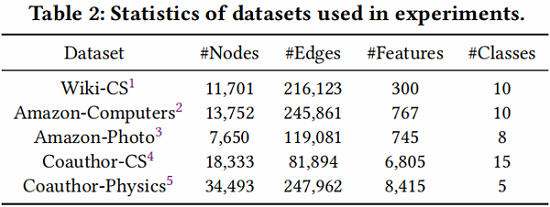基线实验：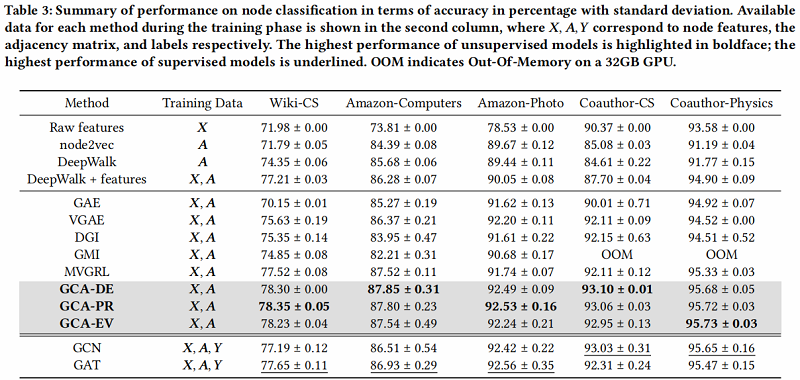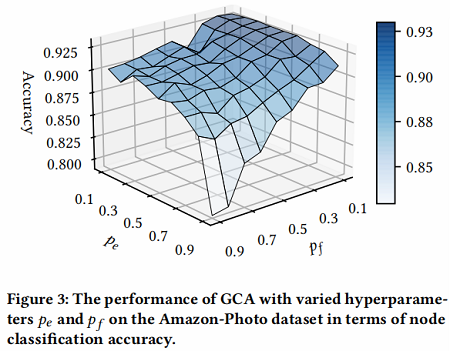# 4 Conclusion

开发了一种自适应数据增强对比学习框架。

2021-04-12 创建文章
2022-06-14 精读